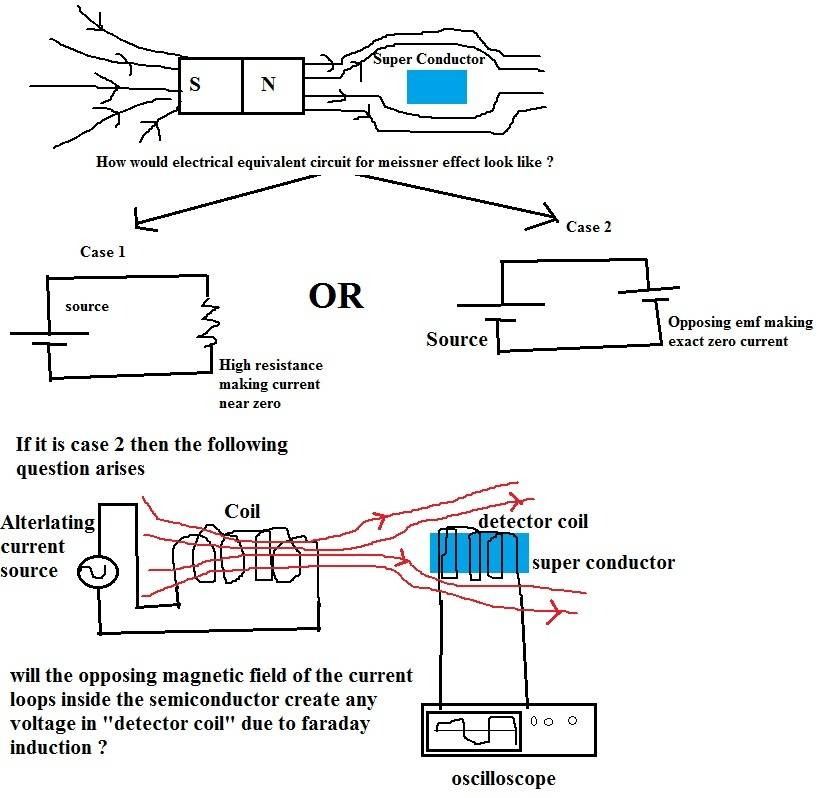# Magnetic Permeability: Temperature Effects

• Narayanan KR

#### Narayanan KR

TL;DR Summary
Is the superconductor in meissner effect able to completely repel external magnetic fields because it provides large magnetic reluctance(small permeability) thereby forcing the external flux to chose alternate paths of low reluctance as shown in case 1 electrical equivalent or is it because an equal and opposite field gets produced by current loops inside the superconductor(T<Tc) as shown by case 2 circuit
If it is case 2 then can this reaction field be harnessed by a coil for electricity ?One more thing i forgot to add, is magnetic permeability of a metal constant irrespective of its temperature including superconducting temperature ?

The Meissner effect can make the magnet float, but the large reluctance seems unable to do this.

In addition, under the same size ratio, the maximum reluctance will be limited by the permeability of free space, unless we artificially reduce the relative permeability to less than 1.

•Narayanan KR
The Meissner effect can make the magnet float, but the large reluctance seems unable to do this.

In addition, under the same size ratio, the maximum reluctance will be limited by the permeability of free space, unless we artificially reduce the relative permeability to less than 1.
so the meissner effect is due to current loops followed by opposing magnetic fields created in the semiconductor ?

so the meissner effect is due to current loops followed by opposing magnetic fields created in the semiconductor ?
It seems that the situation is not far from what you said.

Can the coil use this reaction field to generate electricity?

In fact, I don't know much about how the Meissner effect works.
But if you want to use it to generate electricity, what do you think of its advantages and where the original energy comes from ?

•Narayanan KR
It seems that the situation is not far from what you said.

In fact, I don't know much about how the Meissner effect works.
But if you want to use it to generate electricity, what do you think of its advantages and where the original energy comes from ?
i guess the opposing field in the semiconductor is equal to external flux but never large enough to allow itself to be detected by a coil, it may have H but B=0